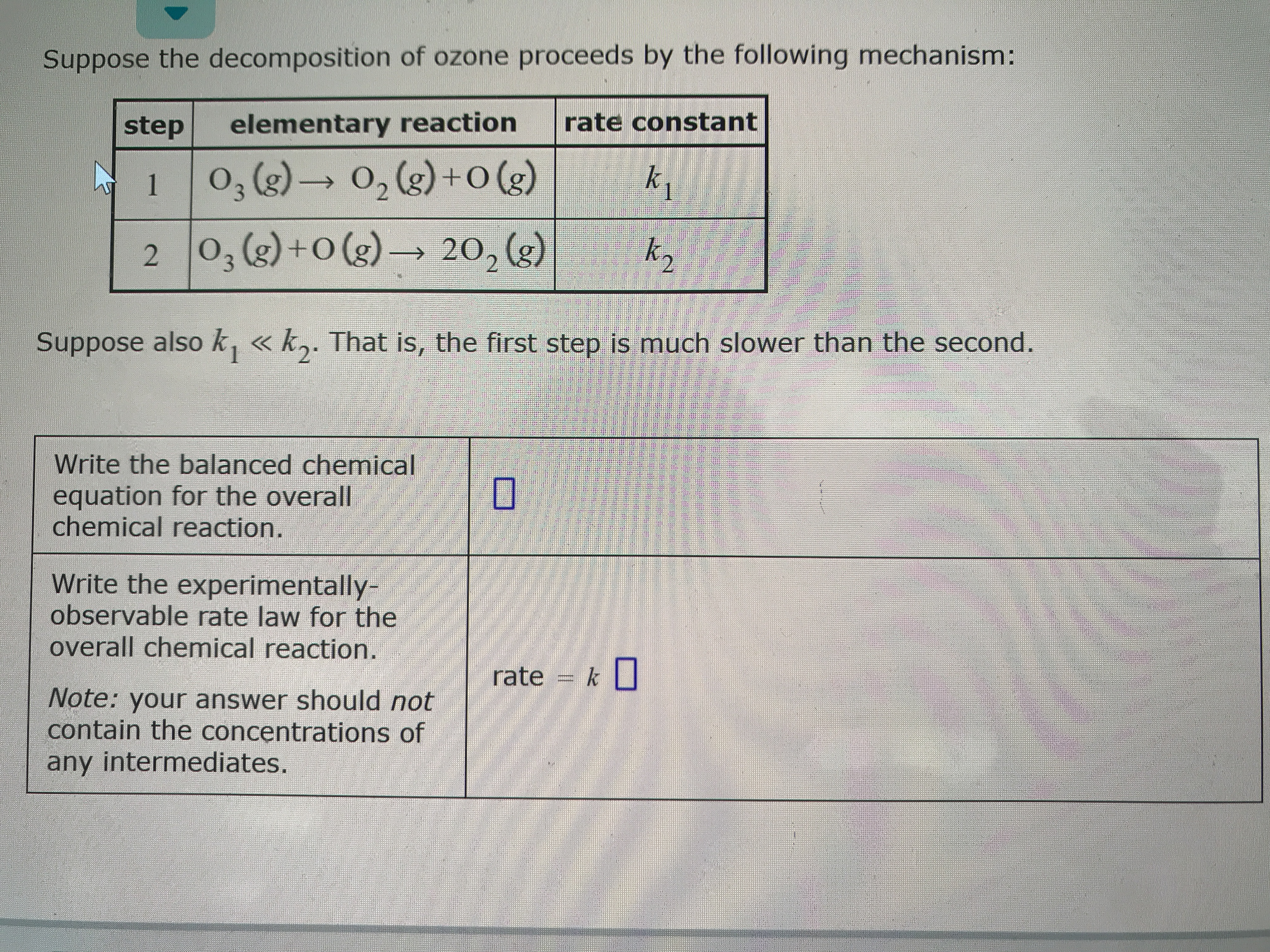# Suppose the decomposition of ozone proceeds by the following mechanism: Step 1, elementary reaction, rate constant 1 , O3 (g) --> O2 (g) + O (g), k1 2, O3 (g) + O (g), --> 2O2 (g), k2 Suppose also k1 << k2. That is the first step is so much slower than the second. Write the balanced chemical equation for the overall chemical reaction Write the experimentally-observable rate law for the overall chemical reaction Note: your answer should not contain the concentrations of any intermediates Writing the rate law implied by a simple mechanism with an initial slope step